# ISEE Upper Level Quantitative : Data Analysis

## Example Questions

### Example Question #3 : Tables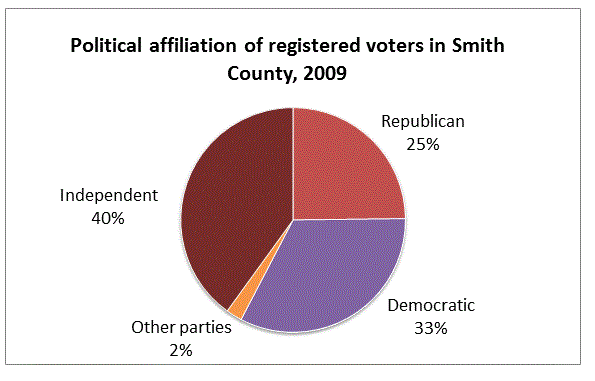Refer to the above diagram. Which is the greater quantity?

(a) The number of registered Republicans in Smith County

(b) 5,000

(a) and (b) are equal

It is impossible to tell from the information given.

(b) is greater

(a) is greater

It is impossible to tell from the information given.

Explanation:

The graph only gives the percent of the population registered in each party, not the number of people, or any way of finding the number of people. It is impossible to determine the number of Republicans in Smith County, so it is also impossible to determine how this number compares to 5,000.

### Example Question #7 : How To Find The Answer From A Table

The following is a portion of the menu at Moonbucks Coffee: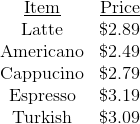Today only, if you buy two espressos at regular price, you can buy any third beverage for $1.00. Which is the greater quantity? (a) The price of two espressos and a cappucino, bought today (b) The price of three espressos, bought today Possible Answers: (b) is greater (a) and (b) are equal It is impossible to tell from the information given (a) is greater Correct answer: (a) and (b) are equal Explanation: In each case, two espressos are being purchased at a regular price, and a third beverage is being purchased at$1.00. This means both sets of drinks cost the same.

### Example Question #4 : Tables

A pair of fair dice are tossed. Which is the greater quantity?

(a) The probability that the sum will be at least eight

(b) The probability that the sum will be at most six

(a) is greater

(b) is greater

(a) and (b) are equal

It is impossible to tell from the information given

(a) and (b) are equal

Explanation:

The following table illustrates the ways that the dice can come up. The orange cells indicate a roll of 6 or less; the pink cells indicate a roll of eight or more.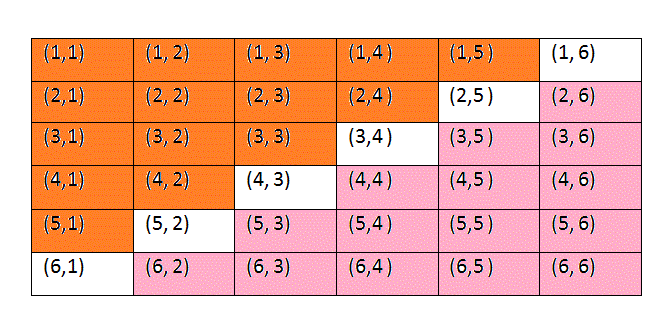Since each of these outcomes are equally likely, and there are fifteen outcomes that are favorable to each of the two events, the probabilities are the same.

### Example Question #1 : Tables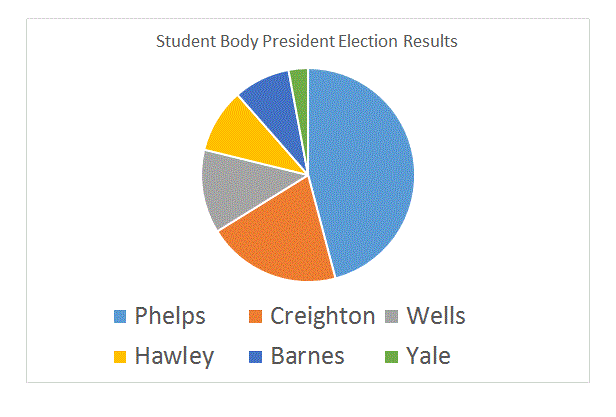Refer to the above circle graph. Which of these quantities is greater?

(a) The number of students who voted for Phelps

(b) The number of students who voted for candidates other than Phelps

It is impossible to tell from the information given.

(b) is greater.

(a) is greater.

(a) and (b) are equal.

(b) is greater.

Explanation:

The portion of the circle graph representing Phelps is light blue; it takes up less than half of the graph, so fewer than half of the votes were won by Phelps. (b) is correct.

### Example Question #10 : How To Find The Answer From A Table

The following is a portion of the menu at Moonbucks Coffee:Which is the greater quantity?

(a) The price of two lattes and a cappucino

(b) The price of two Americanos and an espresso

It is impossible to tell from the information given.

(a) and (b) are equal.

(b) is greater.

(a) is greater.

(a) is greater.

Explanation:(a) Add: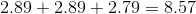(b) Add: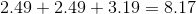(a) is the greater quantity.

### Example Question #21 : Data Analysis

A pair of fair dice are tossed. Which is the greater quantity?

(a) The probability that a 6, a 7, or an 8 will come up

(b) The probability that a number other than 6, 7, or 8 will come up

(b) is greater.

It is impossible to tell from the information given.

(a) is greater.

(a) and (b) are equal.

(b) is greater.

Explanation:

Out of thirty-six possible rolls, there are five ways to roll a six: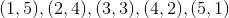Six ways to roll a seven: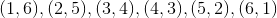Five ways to roll an eight: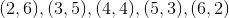This is sixteen rolls out of thirty-six. Therefore, there are twenty rolls that do not result in one of these three numbers. Since all rolls are equally likely, this makes (b) the greater probability.

### Example Question #22 : Data Analysis And Probability

The following is a portion of the menu at Moonbucks Coffee:Which is the greater quantity?

(a) The price of two lattes and a large milk

(b) The price of two espressos and a large milk

It is impossible to tell from the information given.

(a) and (b) are equal.

(a) is greater.

(b) is greater.

(b) is greater.

Explanation:

Although the price of the large milk is not given, it is not needed here since one large milk appears in both orders. All that is needed are the prices of the lattes and the espressos. Since an espresso costs more than a latte, two espressos and a milk cost more than two lattes and a milk. This makes (b) greater.

### Example Question #23 : Data Analysis And Probability

The following is a portion of the menu at Moonbucks Coffee:Today only, if you purchase two Turkish coffees, you get the third one for.

Which is the greater quantity?

(a) The price of three Turkish coffees, bought today

(b) The price of three Americanos.

It is impossible to tell from the information given.

(a) and (b) are equal.

(a) is greater.

(b) is greater.

(a) is greater.

Explanation:

(a) The three Turkish coffees cost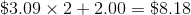.

(b) The three Americanos cost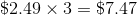.

(a) is the greater quantity.

### Example Question #11 : How To Find The Answer From A Table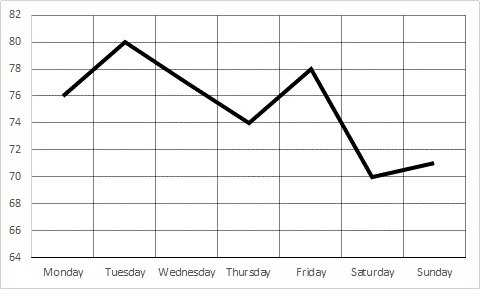Refer to the above graph, which shows the high temperatures in the town of Smithville over seven days.

Which is the greater quantity?

(a) The high temperature for Sunday

(b) The low temperature for Saturday

(b) is greater.

It is impossible to tell from the information given.

(a) and (b) are equal.

(a) is greater.

(a) is greater.

Explanation:

From the graph, it can be seen that the high temperature for Sunday is higher that the high temperature for Saturday, which, by definition, must be greater than the low temperature for Saturday. That makes (a) greater.

### Example Question #12 : How To Find The Answer From A Table

The following is a portion of the menu at Moonbucks Coffee:Assume there is no tax on beverages.

Steve bought a Turkish coffee and paid for it with a five dollar bill. Marsha bought three cafe Americanos and paid for them with a ten-dollar bill. Which is the greater quantity?

(a) The amount of change Steve got back

(b) The amount of change Marsha got back

(a) and (b) are equal.

It is impossible to tell from the information given.

(b) is greater.

(a) is greater.

(b) is greater.

Explanation:

(a) Steve paid $3.09 for his Turkish coffee. Since he paid$5.00, his change was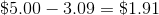.

(b) Marsha paidfor her three Americanos. Since she paid \$10.00, her change was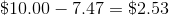.

Marsha got more change back, making (b) higher.

### All ISEE Upper Level Quantitative Resources